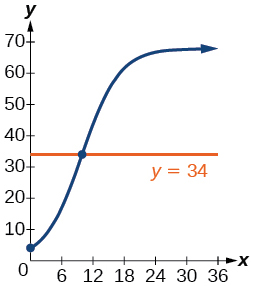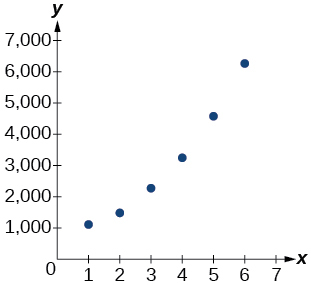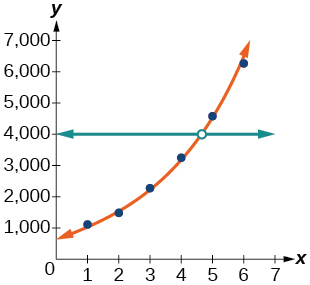# 4.8 Fitting exponential models to data  (Page 7/12)

 Page 7 / 12

What might a scatterplot of data points look like if it were best described by a logarithmic model?

What does the y -intercept on the graph of a logistic equation correspond to for a population modeled by that equation?

The y -intercept on the graph of a logistic equation corresponds to the initial population for the population model.

## Graphical

For the following exercises, match the given function of best fit with the appropriate scatterplot in [link] through [link] . Answer using the letter beneath the matching graph.

$y=10.209{e}^{-0.294x}$

$y=5.598-1.912\mathrm{ln}\left(x\right)$

C

$y=2.104{\left(1.479\right)}^{x}$

$y=4.607+2.733\mathrm{ln}\left(x\right)$

B

$y=\frac{14.005}{1+2.79{e}^{-0.812x}}$

## Numeric

To the nearest whole number, what is the initial value of a population modeled by the logistic equation $\text{\hspace{0.17em}}P\left(t\right)=\frac{175}{1+6.995{e}^{-0.68t}}?\text{\hspace{0.17em}}$ What is the carrying capacity?

$P\left(0\right)=22\text{\hspace{0.17em}}$ ; 175

Rewrite the exponential model $\text{\hspace{0.17em}}A\left(t\right)=1550{\left(1.085\right)}^{x}\text{\hspace{0.17em}}$ as an equivalent model with base $\text{\hspace{0.17em}}e.\text{\hspace{0.17em}}$ Express the exponent to four significant digits.

A logarithmic model is given by the equation $\text{\hspace{0.17em}}h\left(p\right)=67.682-5.792\mathrm{ln}\left(p\right).\text{\hspace{0.17em}}$ To the nearest hundredth, for what value of $\text{\hspace{0.17em}}p\text{\hspace{0.17em}}$ does $\text{\hspace{0.17em}}h\left(p\right)=62?$

$p\approx 2.67$

A logistic model is given by the equation $\text{\hspace{0.17em}}P\left(t\right)=\frac{90}{1+5{e}^{-0.42t}}.\text{\hspace{0.17em}}$ To the nearest hundredth, for what value of t does $\text{\hspace{0.17em}}P\left(t\right)=45?$

What is the y -intercept on the graph of the logistic model given in the previous exercise?

y -intercept: $\text{\hspace{0.17em}}\left(0,15\right)$

## Technology

For the following exercises, use this scenario: The population $\text{\hspace{0.17em}}P\text{\hspace{0.17em}}$ of a koi pond over $\text{\hspace{0.17em}}x\text{\hspace{0.17em}}$ months is modeled by the function $\text{\hspace{0.17em}}P\left(x\right)=\frac{68}{1+16{e}^{-0.28x}}.$

Graph the population model to show the population over a span of $\text{\hspace{0.17em}}3\text{\hspace{0.17em}}$ years.

What was the initial population of koi?

$4\text{\hspace{0.17em}}$ koi

How many koi will the pond have after one and a half years?

How many months will it take before there are $\text{\hspace{0.17em}}20\text{\hspace{0.17em}}$ koi in the pond?

about $\text{\hspace{0.17em}}6.8\text{\hspace{0.17em}}$ months.

Use the intersect feature to approximate the number of months it will take before the population of the pond reaches half its carrying capacity.For the following exercises, use this scenario: The population $\text{\hspace{0.17em}}P\text{\hspace{0.17em}}$ of an endangered species habitat for wolves is modeled by the function $\text{\hspace{0.17em}}P\left(x\right)=\frac{558}{1+54.8{e}^{-0.462x}},$ where $\text{\hspace{0.17em}}x\text{\hspace{0.17em}}$ is given in years.

Graph the population model to show the population over a span of $\text{\hspace{0.17em}}10\text{\hspace{0.17em}}$ years.

What was the initial population of wolves transported to the habitat?

$10\text{\hspace{0.17em}}$ wolves

How many wolves will the habitat have after $\text{\hspace{0.17em}}3\text{\hspace{0.17em}}$ years?

How many years will it take before there are $\text{\hspace{0.17em}}100\text{\hspace{0.17em}}$ wolves in the habitat?

Use the intersect feature to approximate the number of years it will take before the population of the habitat reaches half its carrying capacity.

For the following exercises, refer to [link] .

 x f(x) 1 1125 2 1495 3 2310 4 3294 5 4650 6 6361

Use a graphing calculator to create a scatter diagram of the data.Use the regression feature to find an exponential function that best fits the data in the table.

Write the exponential function as an exponential equation with base $\text{\hspace{0.17em}}e.$

$f\left(x\right)=776.682{e}^{0.3549x}$

Graph the exponential equation on the scatter diagram.

Use the intersect feature to find the value of $\text{\hspace{0.17em}}x\text{\hspace{0.17em}}$ for which $\text{\hspace{0.17em}}f\left(x\right)=4000.$

When $\text{\hspace{0.17em}}f\left(x\right)=4000,$ $x\approx 4.6.$For the following exercises, refer to [link] .

 x f(x) 1 555 2 383 3 307 4 210 5 158 6 122

Use a graphing calculator to create a scatter diagram of the data.

what is set?
a colony of bacteria is growing exponentially doubling in size every 100 minutes. how much minutes will it take for the colony of bacteria to triple in size
I got 300 minutes. is it right?
Patience
no. should be about 150 minutes.
Jason
It should be 158.5 minutes.
Mr
ok, thanks
Patience
100•3=300 300=50•2^x 6=2^x x=log_2(6) =2.5849625 so, 300=50•2^2.5849625 and, so, the # of bacteria will double every (100•2.5849625) = 258.49625 minutes
Thomas
what is the importance knowing the graph of circular functions?
can get some help basic precalculus
What do you need help with?
Andrew
how to convert general to standard form with not perfect trinomial
can get some help inverse function
ismail
Rectangle coordinate
how to find for x
it depends on the equation
Robert
yeah, it does. why do we attempt to gain all of them one side or the other?
Melissa
whats a domain
The domain of a function is the set of all input on which the function is defined. For example all real numbers are the Domain of any Polynomial function.
Spiro
Spiro; thanks for putting it out there like that, 😁
Melissa
foci (–7,–17) and (–7,17), the absolute value of the differenceof the distances of any point from the foci is 24.
difference between calculus and pre calculus?
give me an example of a problem so that I can practice answering
x³+y³+z³=42
Robert
dont forget the cube in each variable ;)
Robert
of she solves that, well ... then she has a lot of computational force under her command ....
Walter
what is a function?
I want to learn about the law of exponent
explain this
what is functions?
A mathematical relation such that every input has only one out.
Spiro
yes..it is a relationo of orders pairs of sets one or more input that leads to a exactly one output.
Mubita
Is a rule that assigns to each element X in a set A exactly one element, called F(x), in a set B.
RichieRich

#### Get Jobilize Job Search Mobile App in your pocket Now!ByByBy John GabrieliBy Anonymous UserBy Stephen VoronBy Melinda SalzerBy Anh DaoBy David CoreyBy Mary MateraBy OpenStaxBy LaToya TrowersBy OpenStax